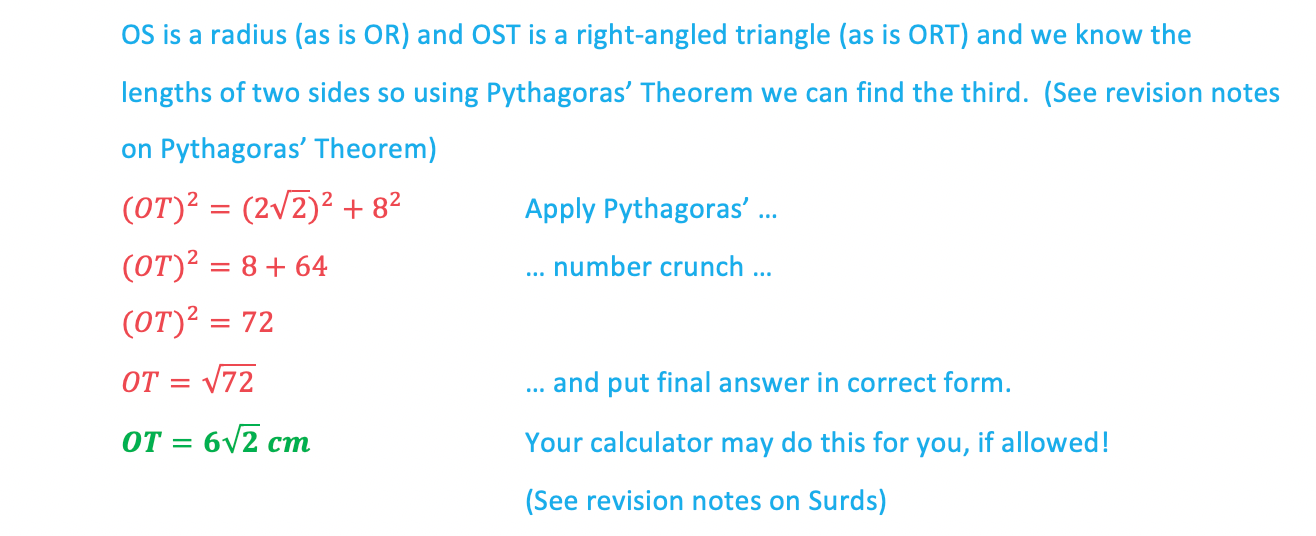# Edexcel IGCSE Maths 复习笔记 4.6.2 Circle Theorems - Tangents

Edexcel IGCSE Maths 复习笔记 4.6.2 Circle Theorems - Tangents

#### What do I need to know about tangents

1. A radius and a tangent are perpendicular• Note that perpendicular lines meet at right angles

2. Not strictly a circle theorem but a very important fact for solving some problems• In this sense the tangents end at two points – the first point is where the two tangents meet and the other end is where each one touches the circle
• Notice because of the circle theorem above that the quadrilateral ROST is a kite with two right angles

#### Exam Tip

Add anything you can to a diagram you have been given – write in any angles and lengths you can work out, even if they don’t seem relevant to the actual question.Also, when asked to “give reasons” (this is very common), aim to quote an angle fact or circle theorem for every angle you find, not just one for the final answer.Likewise, for each angle you work out, try to assign an angle fact or circle theorem to it – giving the names/titles/phrases for each of these is exactly what the examiners want to see.

#### Worked Example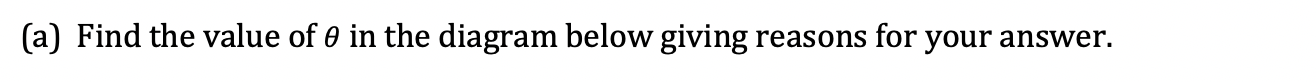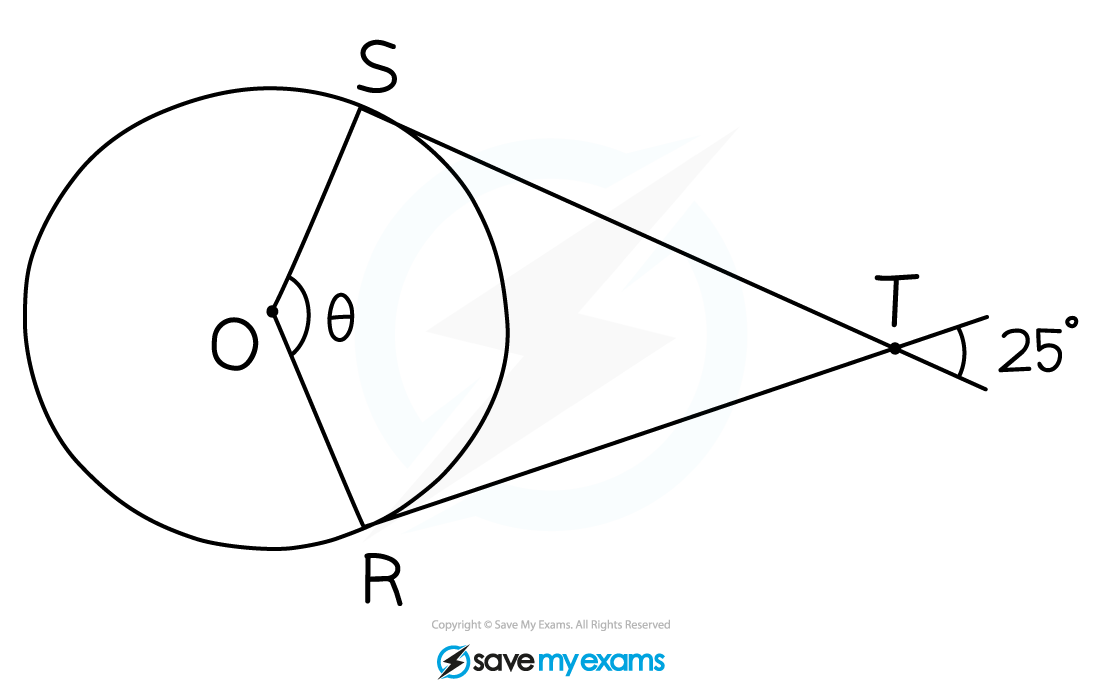#### Worked Example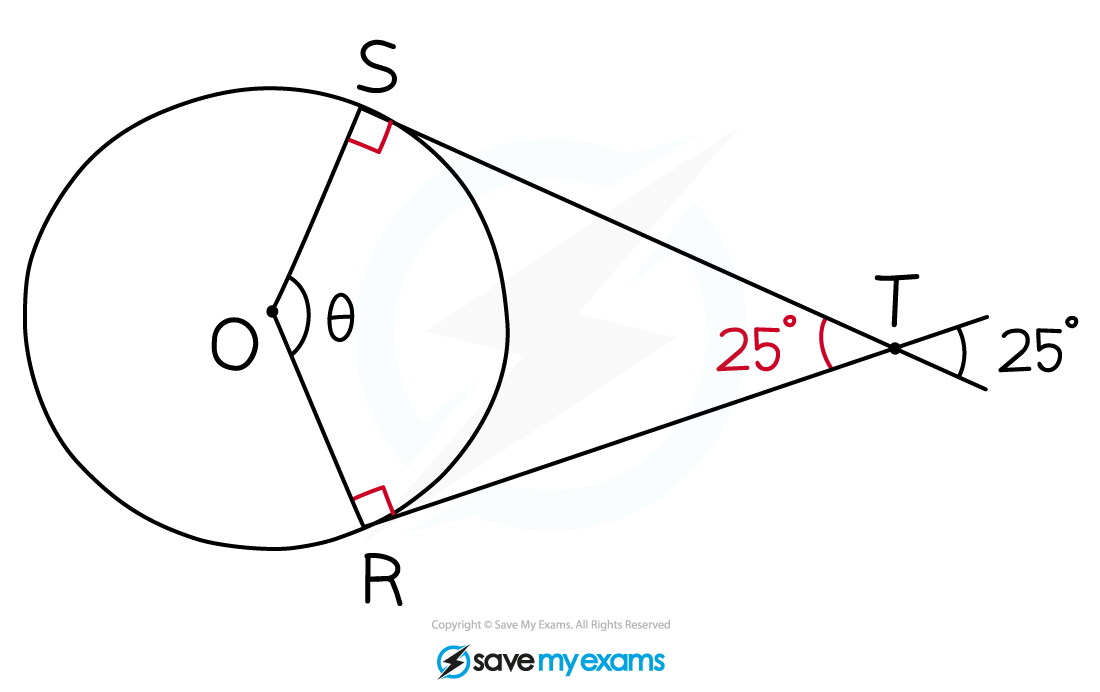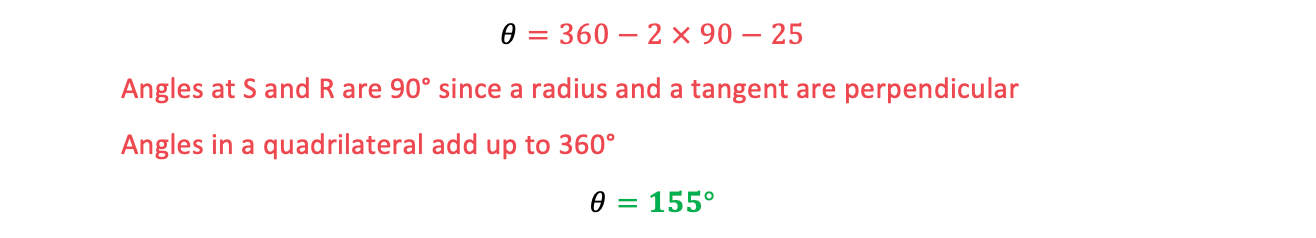#### Worked Example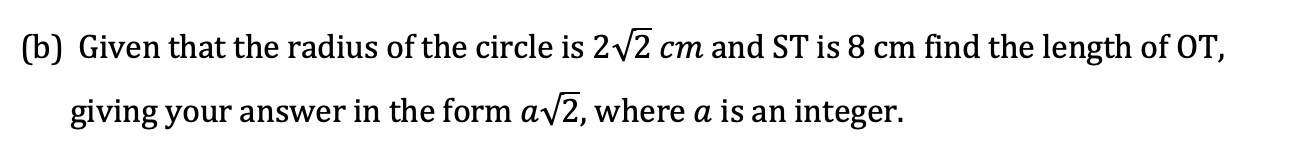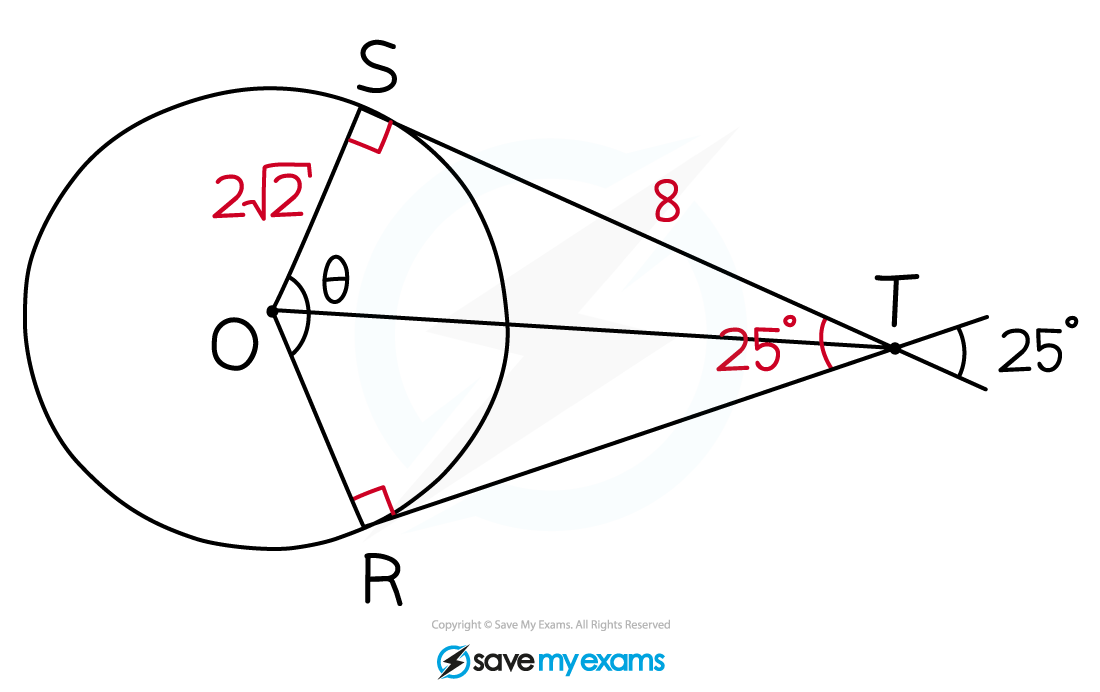#### Worked Example